# KSEEB Solutions for Class 7 Maths Chapter 6 The Triangles and Its Properties Ex 6.1

Students can Download Chapter 6 The Triangles and Its Properties Ex 6.1, Question and Answers, Notes Pdf, KSEEB Solutions for Class 7 Maths, Karnataka State Board Solutions help you to revise complete Syllabus and score more marks in your examinations.

## Karnataka State Syllabus Class 7 Maths Chapter 6 The Triangles and Its Properties Ex 6.1

Question 1.
In ∆PQR, D is the mid-point of $$\overline{\mathbf{Q R}}$$ $$\overline{\mathbf{P M}}$$ is ___
Solution:
$$\overline{\mathbf{P M}}$$ is the altitude of the ∆PQR
∆ PQR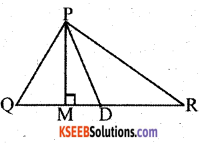$$\overline{\mathbf{P D}}$$ is ___.
$$\overline{\mathbf{P D}}$$ is the median of the ∆ PQR
Is QM = MR?
No, QM ≠ MR ?Question 2.
Draw rough sketches for the following:
Solution:
a) In ∆ ABC, BE is a median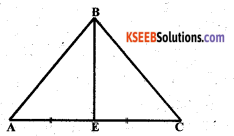b) In ∆ PQR, PQ and PR are altitudes of the triangle.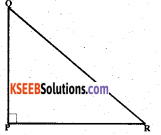c) In ∆XYZ, YL is an altitude in the exterior of the triangle.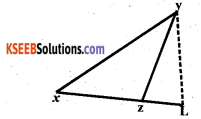Question 3.
Verify by drawing a diagram if the median and altitude of an isosceles triangle can be same.
Solution: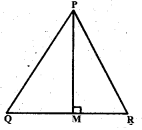In the ∆PQR
PQ = PR
PM is the Median (∵ QM = MR)
PM is the altitude. (∵ PMR = 90°)
∴ In this isosceles triangle median and altitude are the same. (proved / verified).